# Oscillating solution

(diff) ← Older revision | Latest revision (diff) | Newer revision → (diff)

A solution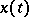of a differential equation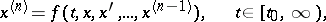(*)

with the property: There exists for any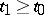a point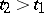such thatchanges sign on passing through it. In many applied problems there arises the question of the existence of an oscillating solution or the question whether all the solutions of equation (*) oscillate. Many sufficient conditions are known under which equation (*) has an oscillating solution (see , , ). For example, any non-trivial solution of the equationwith constant coefficients is oscillating if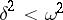; every non-trivial solution of the equation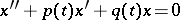with-periodic coefficients is oscillating if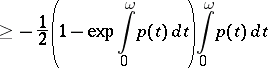and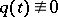on.

In a number of applications there arises the question of the existence of oscillating solutions (in the above sense) of a system of ordinary differential equations. For example, in control theory one studies the oscillation relative to a given hyperplane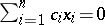of the solutions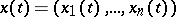of the system of equations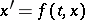, that is, the question whether the function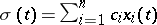oscillates.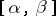-oscillating solutions are also studied; a bounded solutionof the systemis called-oscillating ifis oscillating and for anythere are points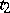andsuch that,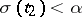,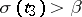, where. For the system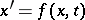there also exist other definitions of an oscillating solution.

How to Cite This Entry:
Oscillating solution. Encyclopedia of Mathematics. URL: http://encyclopediaofmath.org/index.php?title=Oscillating_solution&oldid=11276
This article was adapted from an original article by Yu.V. KomlenkoE.L. Tonkov (originator), which appeared in Encyclopedia of Mathematics - ISBN 1402006098. See original article